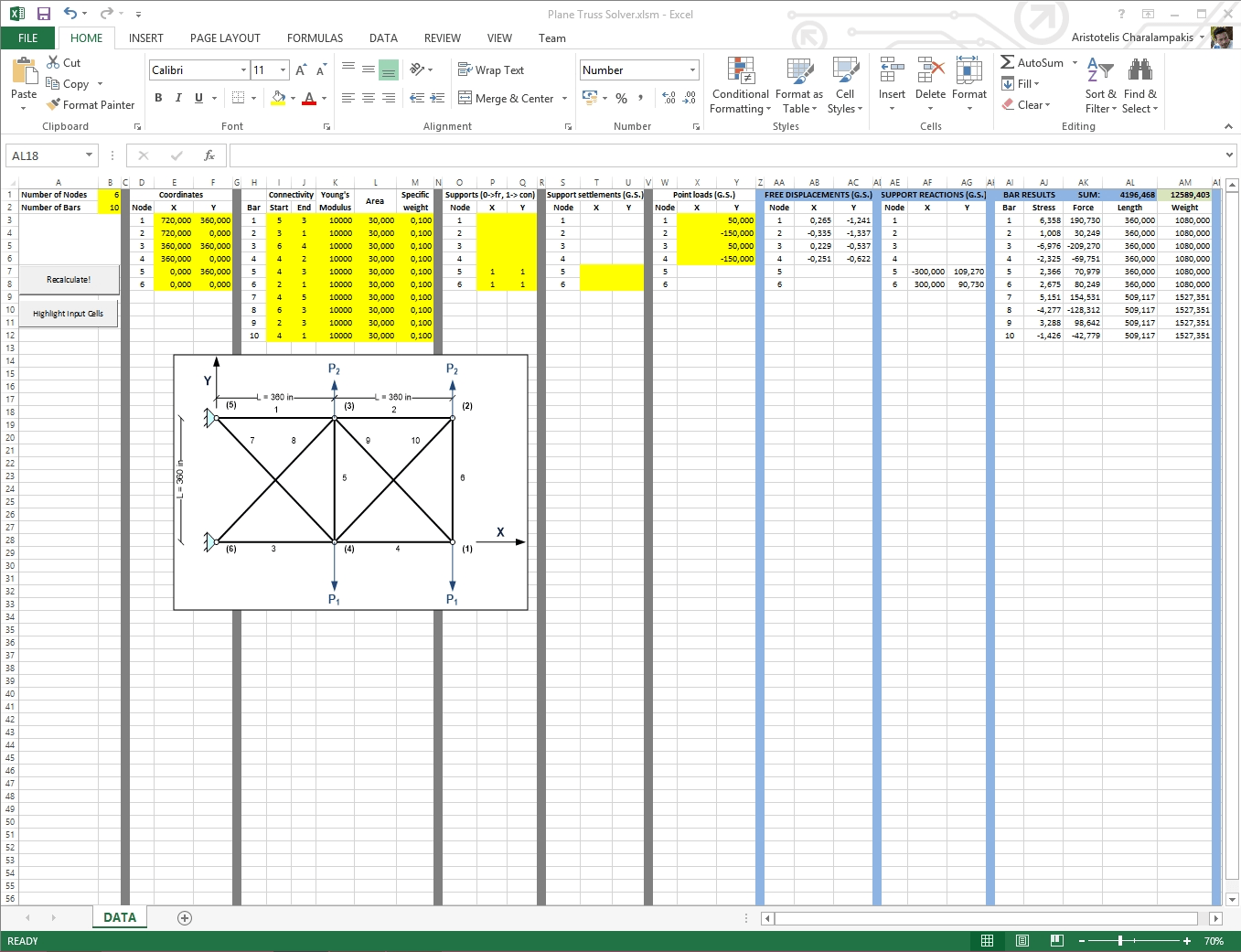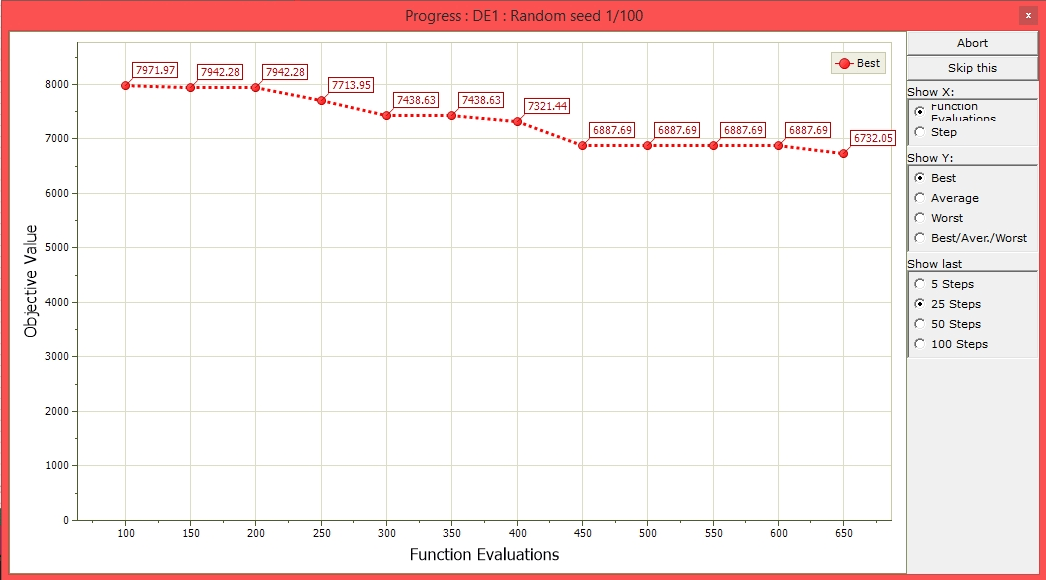## Introduction

The purpose of this example is the optimization (minimization) of the weight of a planar truss using both Microsoft Excel cell functions and VBA (Visual Basic for Applications).

VBA is a versatile tool which extends the capabilities of Microsoft Excel considerably. You can refer to a very simple example, the Sphere function, to see how to set up a spreadsheet with VBA.

In this example, we have created a spreadsheet which solves planar trusses. As an application, a truss is optimized which consists of 6 nodes and 10 bars (members).

## Step 1 : Setting-up the spreadsheet

We have already created the spreadsheet, which can be found here. It looks like this:After modifying certain cells, such as the number of nodes or the number of bars, you can use the "Highlight Input Cells" button to highlight the cells that need input.

You can hit the "Recalculate" button to analyze the truss based on the present data.

In order to take into account the restriction on the number of variables imposed by the demo version, we have arranged the variables into groups of two: bar 1 and 2 share the same area, bar 3 and 4, etc. Of course, there is no such restriction in the full version.

A consistent unit system is used; in this example distances are measured in inches, the forces in kips and the weight in lbs. The supports are indicated per degree-of-freedom (dof); 1 means a constrained dof and nothing or zero stands for a free dof. Also, in this example, P1 = 150 kips and P2 = 50 kips, as shown in the picture.

The weight of the truss is evaluated in cell AM1. We wish to minimize this value, therefore we set the objective function to take values from this cell. The minimization must take into account certain constraints. In this example, the stress of each bar (cells AJ3 to AJ12) must take values in the range [-25, 25] (ksi). Also, all node displacements (cells AB3 to AC6) must take values in the range [-2, 2] (in). We have included all these constraints in xlOptimizer. Any violation adds the penalty function 1000*X + 1000 to the weight of a candidate design, where X is the absolute value of the violation. Alternatively, you could easily implement the penalty functions in the spreadsheet; this is actually faster, because it minimizes the data transfer between xlOptimizer and Microsoft Excel

## Step 2 : Minimization

After setting up an algorithm, in this case the Differential Evolution, we can begin the minimization:That's it!

The files for the example described in this article can be here found here.